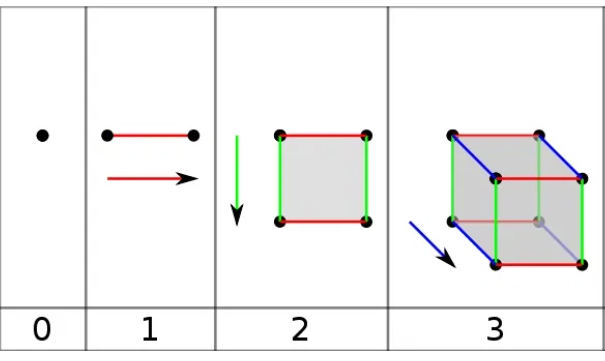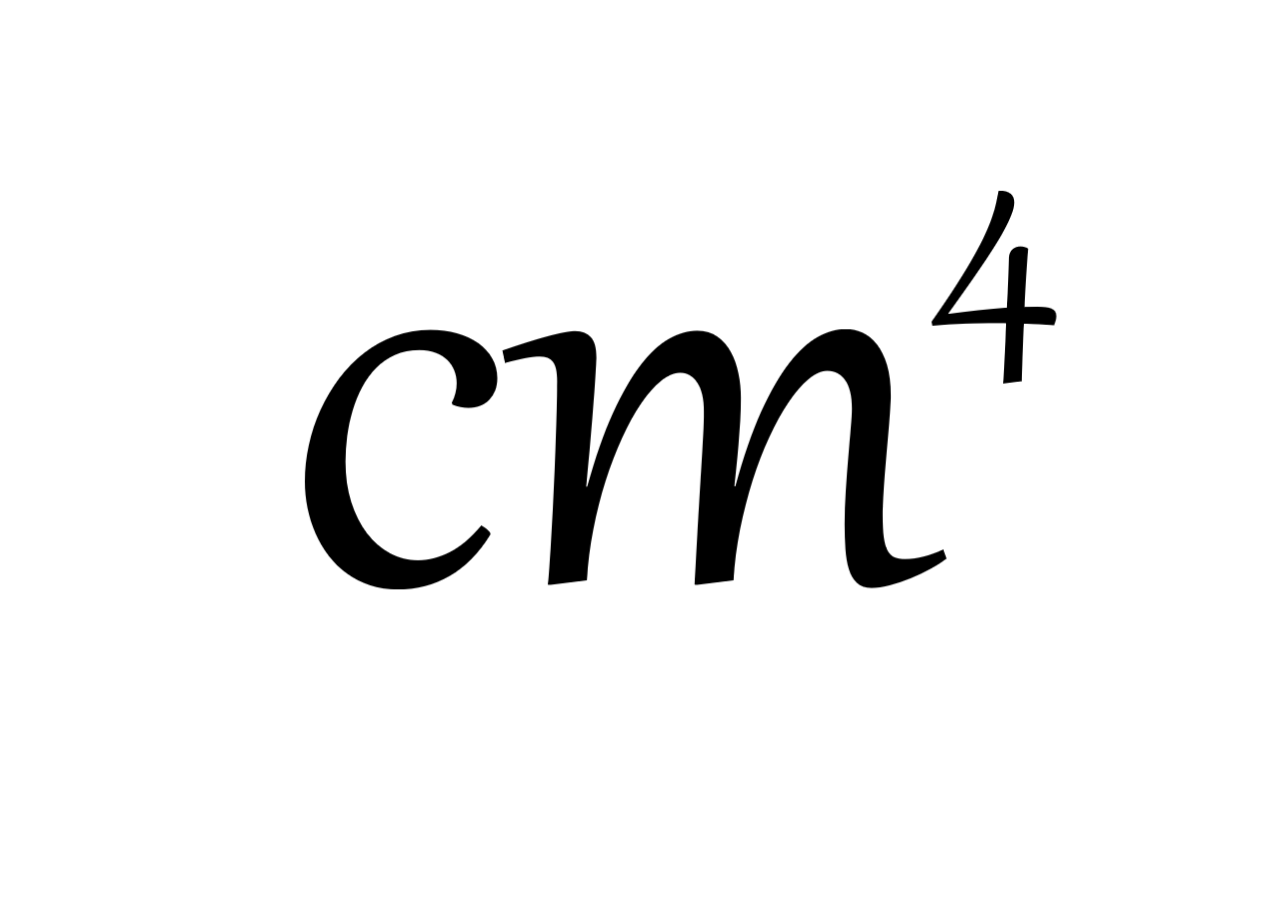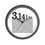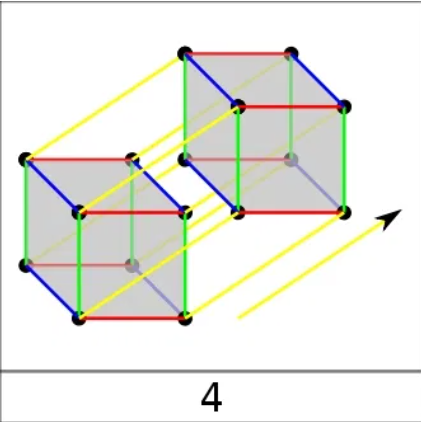# 4th dimension & Non-euclidean geometry (Part 1)

I'm currently interested in the concept of high dimensions and stuff, I did many types of research, mostly games Devlogs which gave great assumption of the higher dimensions. I'll share what I learned in a series of notes.

First, of course, what is the 4th dimension? Some believed that the 4th dimension is the axis of time, but I'm going to talk about another concept here. Let's take a look at some lower dimensions:0D: 0d objects have no axis to exist on, almost equals to nothing, but there is an existing object. (We can't see)

1D: Objects can exist on the x-axis, but no others, space took up by the object is called length. (We can't see)

2D: Objects can exist on the x and y-axis, a plane! Y-axis is perpendicular to the x-axis, space took up is called the area.

3D: Objects can exist on the x, y and z-axis. Z-axis is perpendicular to both x and y-axis, space took up is called the volume.

Now, some brainstorms?

(Concept) 4D: Objects can exist on the x, y, z and w-axis. W-axis is perpendicular to all x, y and z-axis ( Can you imagine? ) We don't have a proper name for an area taken up by a 4d object, but let's say if all 4 axis is measured in centimetres, then our unit for the object will be...In the next part, we'll discuss how will 4d object look like.

And feel free to comment if you have any ideas!Note by Half Pass3
3 months ago

This discussion board is a place to discuss our Daily Challenges and the math and science related to those challenges. Explanations are more than just a solution — they should explain the steps and thinking strategies that you used to obtain the solution. Comments should further the discussion of math and science.

When posting on Brilliant:

• Use the emojis to react to an explanation, whether you're congratulating a job well done , or just really confused .
• Ask specific questions about the challenge or the steps in somebody's explanation. Well-posed questions can add a lot to the discussion, but posting "I don't understand!" doesn't help anyone.
• Try to contribute something new to the discussion, whether it is an extension, generalization or other idea related to the challenge.

MarkdownAppears as
*italics* or _italics_ italics
**bold** or __bold__ bold
- bulleted- list
• bulleted
• list
1. numbered2. list
1. numbered
2. list
Note: you must add a full line of space before and after lists for them to show up correctly
paragraph 1paragraph 2

paragraph 1

paragraph 2

[example link](https://brilliant.org)example link
> This is a quote
This is a quote
    # I indented these lines
# 4 spaces, and now they show
# up as a code block.

print "hello world"
# I indented these lines
# 4 spaces, and now they show
# up as a code block.

print "hello world"
MathAppears as
Remember to wrap math in $$ ... $$ or $ ... $ to ensure proper formatting.
2 \times 3 $2 \times 3$
2^{34} $2^{34}$
a_{i-1} $a_{i-1}$
\frac{2}{3} $\frac{2}{3}$
\sqrt{2} $\sqrt{2}$
\sum_{i=1}^3 $\sum_{i=1}^3$
\sin \theta $\sin \theta$
\boxed{123} $\boxed{123}$

Sort by:

BTW this is an illustration of a 4d cube which I don't quite agree on:- 3 months ago

What about an animation? Check Wikipedia's animation of a $4$D cube.

- 3 months ago

yeah, This concept doesn't quite fit that gif too, but I'll definitely add some animations in the next part!

- 3 months ago

You might like this: